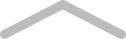# Introduction

This book is going to pretty maths-dependant, so be warned. This book assumes no prior knowledge of physics, and we will start learning about classical physics before moving on to relativistic and quantum physics. I have organised this book in a way that I find intuitive, not in chronological order or in order of difficulty. I tried to make the chapters flow as well as I could. So here is how this book is organised:

• Introduction
• Basics

CLASSICAL PHYSICS

Classical physics contains all of physics from before the 20th Century.

• Mechanics
• Waves/Oscillations
• Thermodynamics
• Electromagnetism
• Optics

MODERN PHYSICS

Modern physics contains all of physics since after around 1905 when Einstein published his Special Theory of Relativity.

• Relativity
• Quantum Mechanics
• Particle Physics
• Astrophysics/Cosmology

And so, on with the introduction. The aim of this introduction is to explain what physics is and how you do physics. Let's start with what physics is.

Physics is the branch of science that deals with the nature and properties of matter and energy.

Now let's talk about how physics is done.

First of all, you observe something happening and make a statement about it e.g the ball is rolling. Then you make a hypothesis that attempts to explain why this occurred e.g the ball is rolling because it was pushed. You then test this hypothesis by creating an experiment and predicting the results e.g we have several balls, some of which will be pushed. We predict that the balls that are pushed will move, and the balls that are not pushed will not move. Now we run the experiment and compare the results to our predictions. In this case our hypothesis turned out to be correct.Log in or Join Tablo to comment on this chapter...

# Basics

Before we go any further, now would probably be a good time to introduce the basics of experimental uncertainties, and some of the maths that will be required pretty soon into the book. This will mean we won't have to go over any of this later on, and this chapter can be used as a reference if you need it.

• Standard Notation
• Units and Measurements
• Errors and Uncertainties
• Algebra
• Logarithms
• Trigonometry and Geometry
• Probability
• Calculus
• Vectors
• Complex numbers
• MatricesLog in or Join Tablo to comment on this chapter...
~
69 mins to go